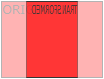# CSS 3D Transforms

## CSS 3D Transforms

CSS also supports 3D transformations.

Mouse over the elements below to see the difference between a 2D and a 3D transformation:

2D rotate
3D rotate

In this chapter you will learn about the following CSS property:

• `transform`

## Browser Support

The numbers in the table specify the first browser version that fully supports the property.

Property
transform 36.0
10.0
16.0
9.0
23.0

## CSS 3D Transforms Methods

With the CSS `transform` property you can use the following 3D transformation methods:

• `rotateX()`
• `rotateY()`
• `rotateZ()`

## The rotateX() MethodThe `rotateX()` method rotates an element around its X-axis at a given degree:

### Example

#myDiv {
transform: rotateX(150deg);
}
Try it Yourself »

## The rotateY() MethodThe `rotateY()` method rotates an element around its Y-axis at a given degree:

### Example

#myDiv {
transform: rotateY(150deg);
}
Try it Yourself »

## The rotateZ() Method

The `rotateZ()` method rotates an element around its Z-axis at a given degree:

### Example

#myDiv {
transform: rotateZ(90deg);
}
Try it Yourself »

## CSS Transform Properties

The following table lists all the 3D transform properties:

Property Description
transform Applies a 2D or 3D transformation to an element
transform-origin Allows you to change the position on transformed elements
transform-style Specifies how nested elements are rendered in 3D space
perspective Specifies the perspective on how 3D elements are viewed
perspective-origin Specifies the bottom position of 3D elements
backface-visibility Defines whether or not an element should be visible when not facing the screen

## CSS 3D Transform Methods

Function Description
matrix3d
(n,n,n,n,n,n,n,n,n,n,n,n,n,n,n,n)
Defines a 3D transformation, using a 4x4 matrix of 16 values
translate3d(x,y,z) Defines a 3D translation
translateX(x) Defines a 3D translation, using only the value for the X-axis
translateY(y) Defines a 3D translation, using only the value for the Y-axis
translateZ(z) Defines a 3D translation, using only the value for the Z-axis
scale3d(x,y,z) Defines a 3D scale transformation
scaleX(x) Defines a 3D scale transformation by giving a value for the X-axis
scaleY(y) Defines a 3D scale transformation by giving a value for the Y-axis
scaleZ(z) Defines a 3D scale transformation by giving a value for the Z-axis
rotate3d(x,y,z,angle) Defines a 3D rotation
rotateX(angle) Defines a 3D rotation along the X-axis
rotateY(angle) Defines a 3D rotation along the Y-axis
rotateZ(angle) Defines a 3D rotation along the Z-axis
perspective(n) Defines a perspective view for a 3D transformed element